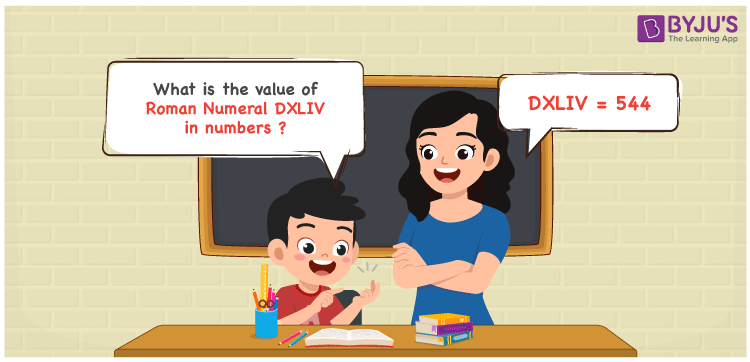Checkout JEE MAINS 2022 Question Paper Analysis : Checkout JEE MAINS 2022 Question Paper Analysis :

# DXLIV Roman Numerals

DXLIV Roman Numerals is 544. Roman Numerals are the system of representing numbers using the Roman alphabet. Download the PDF of Roman Numerals 1 to 1000  for a better understanding of how to express Roman letters for the natural numbers from 1 to 1000 in an effective way. In this article, we will learn how to convert DXLIV Roman Numeral into numbers with a complete explanation.

 Number Roman Numeral 544 DXLIV

## How to Write DXLIV Roman Numerals?The following methods help us to represent the numerical value of DXLIV Roman Numerals

First Method:

• Break the Roman Numerals into single letters
• DXLIV = D + (L – X) + (V – I)
• Write the numerical value of each letter and add/subtract them
• DXLIV = 500 + (50 – 10) + (5 – 1) = 544

Second Method:

• In this method, we consider the groups of Roman Numerals for addition or subtraction
• DXLIV = D + XL + IV = 500 + 40 + 4 = 544
• Therefore the numerical value of DXLIV Roman Numerals is 544

## Video Lesson on Roman Numerals## Related Article

Roman Numerals

Roman Numerals 1 to 50

Roman Numerals 1 to 100

Roman Numerals Conversion

L Roman Numerals

Roman Numeral XXXIX

## Frequently Asked Questions on DXLIV Roman Numerals

### What is the numerical value of DXLIV Roman Numerals?

The numerical value of DXLIV Roman Numerals is 544.

### What is the difference between 544 and 200 in Roman Numerals?

544 – 200 = 344. The number 344 in Roman Numerals is CCCXLIV. Hence the difference between 544 and 200 in Roman Numerals is CCCXLIV.

### How do you read the Roman Numeral DXLIV in English?

The Roman Numeral DXLIV is 544 and it is read as Five Hundred Forty-Four in English.